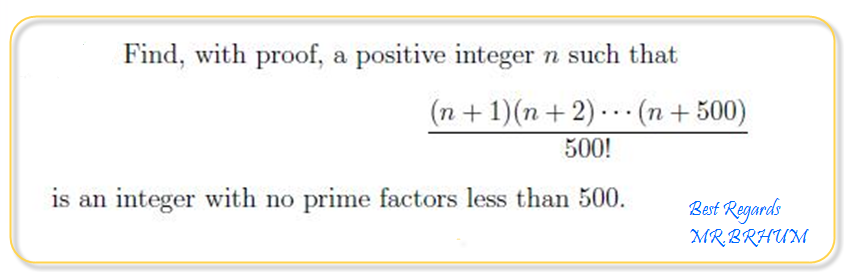Algebra Pre-Algebra and Basic Algebra Math Forum

 June 12th, 2014, 07:57 AM #1 Member   Joined: Jun 2014 From: Math Forum Posts: 67 Thanks: 4 Problem 3Thanks from raul21June 12th, 2014, 05:38 PM #2 Math Team   Joined: Apr 2010 Posts: 2,780 Thanks: 361 Interesting question! It asks for n > 1 such that $\displaystyle {n + 500 \choose 500}$ has no primefactors less than 500. Values of n such that $\displaystyle {n \choose k}$ has no primefactors less than k aren't as such in OEIS yet. Here are values for k = 1 to 30: Code: 1,2 2,3 3,7 4,5 5,23 6,7 7,143 8,44 9,159 10,11 11,47 12,13 13,2239 14,239 15,719 16,17 17,5849 18,19 19,2099 20,43196 21,14871 22,23 23,35423 24,193049 25,2105 26,36287 27,1119 28,29 29,240479 30,31 Would you like to add the sequence?June 12th, 2014, 06:55 PM #3 Math Team   Joined: Oct 2011 From: Ottawa Ontario, Canada Posts: 14,597 Thanks: 1039 Well, don't know what's being asked... However, for [(n+1)(n+2)....(n+500)] / 500! solution is: (n + 500)! / (500! * n!)June 13th, 2014, 03:17 AM #4 Math Team   Joined: Apr 2010 Posts: 2,780 Thanks: 361 Maybe with some examples you see it; If n = 1, then $\displaystyle [(n+1)(n+2)....(n+500)] / 500! = {501 \choose 500} = 501$ Factoring 501 gives 3*167, which has primefactors less than 500. If n = 2 then $\displaystyle [(n+1)(n+2)....(n+500)] / 500! = 125751$ Factoring 125751 gives 3 * 167 * 251 which has primefactors less than 500. ... If n = m then $\displaystyle [(m+1)(m+2)....(m+500)] / 500! = {m + 500\choose 500}$. Factoring $\displaystyle {m + 500\choose 500}$ gives $\displaystyle \cdots$ which has no primefactors less than 500. What is the least possible positive integer value of m?June 13th, 2014, 10:51 AM   #5
Math Team

Joined: Oct 2011

Posts: 14,597
Thanks: 1039

Quote:
 Originally Posted by HoempaIf n = 2 then $\displaystyle [(n+1)(n+2)....(n+500)] / 500! = 125751$
(n + 500)! / (500! * n!)
= (2 + 500)! / (500! * 2!)
= 502! / (500! * 2)
= 125751

All I was doing is providing an equation to get the TOTAL result...June 14th, 2014, 07:31 AM #6 Senior Member   Joined: Dec 2013 From: some subspace Posts: 212 Thanks: 72 Math Focus: real analysis, vector analysis, numerical analysis, discrete mathematics All right. I'm definitely not a person who knows the number theory. But I searched this via google, and I was able to find this .pdf article. On the page 3 (or 205) of the paper, the writer says that if $\displaystyle n = s + m\cdot \left( s! \right)^2$, where $\displaystyle m$ is integer, then $\displaystyle \binom{n} {s}$ has not prime factors less than $\displaystyle s$. So. In case of $\displaystyle \binom{n+500}{500}$ if one choose $\displaystyle n = \left( 500! \right)^2$ then the condition should apply. To test this, I wrote a short python program that prints the smallest prime factor of the binomial coefficient: Code: def binomc(n, k): #binomial coefficient n over k result = 1 for i in range(1, k+1): result = result * (n-i+1) / i return result def smallestfact(l): #finds the smallest factor of l fac = 2 while True: if l % fac == 0: #if l is divisable by fac return fac else: fac += 1 def fact(l): #factorial of l f = 1 vv = 2 while vv <= l: f *= vv vv += 1 return f v = fact(500) v = v*v b = binomc(v+500,500) a = smallestfact(b) print a The output of this program was 503, so it seems that this is some kind of solution. Althought I'm not quite convinced about it. For example, what Hoempa showed earlier, there are much much smaller numbers that could satisfy the condition. For example $\displaystyle \binom{n + 5}{5}$ for which Hoempa found $\displaystyle n = 18$ instead of this approach $\displaystyle n = 14400$. Perhaps someone who knows this kind of things better could clarify?Tags problemThread ToolsShow Printable VersionEmail this Page Display ModesLinear ModeSwitch to Hybrid ModeSwitch to Threaded Mode

 Contact - Home - Forums - Cryptocurrency Forum - Top